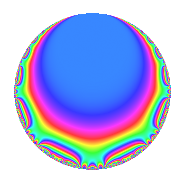# Properties

 Label 336.2.b.bLevel 336 Weight 2 Character orbit 336.b Analytic conductor 2.683 Analytic rank 0 Dimension 2 CM No Inner twists 2

# Related objects

## Newspace parameters

 Level: $$N$$ = $$336 = 2^{4} \cdot 3 \cdot 7$$ Weight: $$k$$ = $$2$$ Character orbit: $$[\chi]$$ = 336.b (of order $$2$$ and degree $$1$$)

## Newform invariants

 Self dual: No Analytic conductor: $$2.68297350792$$ Analytic rank: $$0$$ Dimension: $$2$$ Coefficient field: $$\Q(\sqrt{-6})$$ Coefficient ring: $$\Z[a_1, \ldots, a_{5}]$$ Coefficient ring index: $$1$$ Sato-Tate group: $\mathrm{SU}(2)[C_{2}]$

## $q$-expansion

Coefficients of the $$q$$-expansion are expressed in terms of $$\beta = \sqrt{-6}$$. We also show the integral $$q$$-expansion of the trace form.

 $$f(q)$$ $$=$$ $$q - q^{3} + \beta q^{5} + ( 1 - \beta ) q^{7} + q^{9} +O(q^{10})$$ $$q - q^{3} + \beta q^{5} + ( 1 - \beta ) q^{7} + q^{9} + \beta q^{11} + 2 \beta q^{13} -\beta q^{15} + \beta q^{17} + 2 q^{19} + ( -1 + \beta ) q^{21} + 3 \beta q^{23} - q^{25} - q^{27} + 6 q^{29} -8 q^{31} -\beta q^{33} + ( 6 + \beta ) q^{35} + 4 q^{37} -2 \beta q^{39} -3 \beta q^{41} -2 \beta q^{43} + \beta q^{45} + 12 q^{47} + ( -5 - 2 \beta ) q^{49} -\beta q^{51} -6 q^{53} -6 q^{55} -2 q^{57} -12 q^{59} + ( 1 - \beta ) q^{63} -12 q^{65} -3 \beta q^{69} + 5 \beta q^{71} -6 \beta q^{73} + q^{75} + ( 6 + \beta ) q^{77} -4 \beta q^{79} + q^{81} -6 q^{85} -6 q^{87} -5 \beta q^{89} + ( 12 + 2 \beta ) q^{91} + 8 q^{93} + 2 \beta q^{95} + 2 \beta q^{97} + \beta q^{99} +O(q^{100})$$ $$\operatorname{Tr}(f)(q)$$ $$=$$ $$2q - 2q^{3} + 2q^{7} + 2q^{9} + O(q^{10})$$ $$2q - 2q^{3} + 2q^{7} + 2q^{9} + 4q^{19} - 2q^{21} - 2q^{25} - 2q^{27} + 12q^{29} - 16q^{31} + 12q^{35} + 8q^{37} + 24q^{47} - 10q^{49} - 12q^{53} - 12q^{55} - 4q^{57} - 24q^{59} + 2q^{63} - 24q^{65} + 2q^{75} + 12q^{77} + 2q^{81} - 12q^{85} - 12q^{87} + 24q^{91} + 16q^{93} + O(q^{100})$$

## Character Values

We give the values of $$\chi$$ on generators for $$\left(\mathbb{Z}/336\mathbb{Z}\right)^\times$$.

 $$n$$ $$85$$ $$113$$ $$127$$ $$241$$ $$\chi(n)$$ $$1$$ $$1$$ $$-1$$ $$-1$$

## Embeddings

For each embedding $$\iota_m$$ of the coefficient field, the values $$\iota_m(a_n)$$ are shown below.

For more information on an embedded modular form you can click on its label.

Label $$\iota_m(\nu)$$ $$a_{2}$$ $$a_{3}$$ $$a_{4}$$ $$a_{5}$$ $$a_{6}$$ $$a_{7}$$ $$a_{8}$$ $$a_{9}$$ $$a_{10}$$
223.1
 − 2.44949i 2.44949i
0 −1.00000 0 2.44949i 0 1.00000 + 2.44949i 0 1.00000 0
223.2 0 −1.00000 0 2.44949i 0 1.00000 2.44949i 0 1.00000 0
 $$n$$: e.g. 2-40 or 990-1000 Significant digits: Format: Complex embeddings Normalized embeddings Satake parameters Satake angles

## Inner twists

Char. orbit Parity Mult. Self Twist Proved
1.a Even 1 trivial yes
28.d Even 1 yes

## Hecke kernels

This newform can be constructed as the intersection of the kernels of the following linear operators acting on $$S_{2}^{\mathrm{new}}(336, [\chi])$$:

 $$T_{5}^{2} + 6$$ $$T_{19} - 2$$